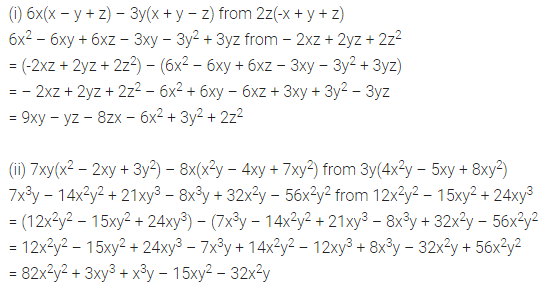# ML Aggarwal Class 8 Solutions for ICSE Maths Chapter 10 Algebraic Expressions and Identities Ex 10.2

## ML Aggarwal Class 8 Solutions for ICSE Maths Chapter 10 Algebraic Expressions and Identities Ex 10.2

Question 1.
Find the product of:
(i) 4x3 and -3xy
(ii) 2xyz and 0
(iii) –$$\frac{2}{3}$$p2q, $$\frac{3}{4}$$pq2 and 5pqr
(iv) -7ab,-3a3 and –$$\frac{2}{7}$$ab2
(v) –$$\frac{1}{2}$$x2 – $$\frac{3}{5}$$xy, $$\frac{2}{3}$$yz and $$\frac{5}{7}$$xyz
Solution: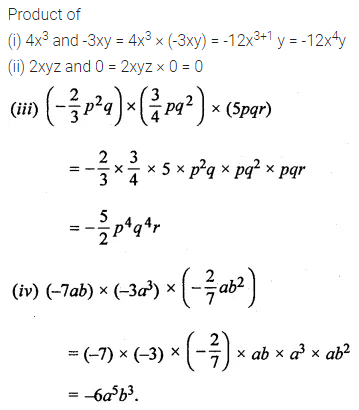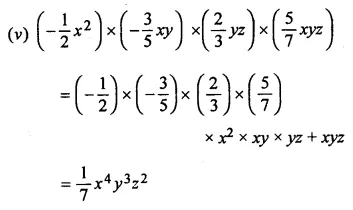Question 2.
Multiply:
(i) (3x – 5y + 7z) by – 3xyz
(ii) (2p2 – 3pq + 5q2 + 5) by – 2pq
(iii) ($$\frac{2}{3}$$a2b – $$\frac{4}{5}$$ab2 + $$\frac{2}{7}$$ab + 3) by 35ab
(iv) (4x2 – 10xy + 7y2 – 8x + 4y + 3) by 3xy
Solution:Question 3.
Find the areas of rectangles with the following pairs of monomials as their lengths and breadths respectively:
(i) (p2q, pq2)
(ii) (5xy, 7xy2)
Solution: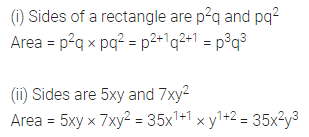Question 4.
Find the volume of rectangular boxes with the following length, breadth and height respectively:
(i) 5ab, 3a2b, 7a4b2
(ii) 2pq, 4q2, 8rp
Solution: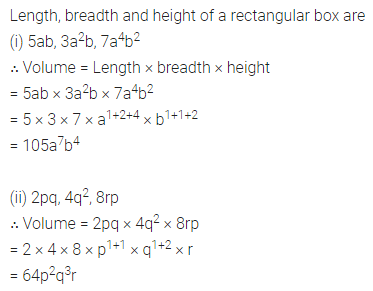Question 5.
Simplify the following expressions and evaluate them as directed:
(i) x2(3 – 2x + x2) for x = 1; x = -1; x = $$\frac{2}{3}$$ and x = –$$\frac{1}{2}$$
(ii) 5xy(3x + 4y – 7) – 3y(xy – x2 + 9) – 8 for x = 2, y = -1
Solution: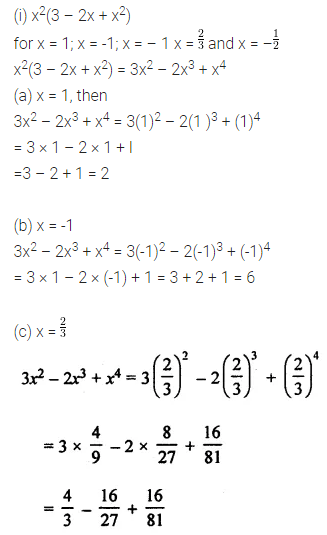Question 6.